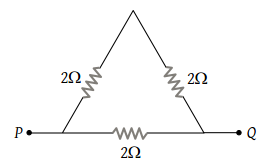NEET Questions Solved

PMT - 1983

Three resistors each of 2 ohm are connected together in a triangular shape. The resistance between any two vertices will be

(1) 4/3 ohm

(2) 3/4 ohm

(3) 3 ohm

(4) 6 ohm

(1) Equivalent resistance of the combination

$=\frac{\left(2+2\right)×2}{2+2+2}=\frac{8}{6}=\frac{4}{3}\Omega$Difficulty Level:

• 60%
• 18%
• 17%
• 7%